Get instant live expert help with Excel or Google Sheets“My Excelchat expert helped me in less than 20 minutes, saving me what would have been 5 hours of work!”

#### Post your problem and you’ll get expert help in seconds.

Your message must be at least 40 characters
Our professional experts are available now. Your privacy is guaranteed.

# Excel ERROR.TYPE FunctionRead time: 20 minutes

The ERROR.TYPE function in Excel returns a number that corresponds to a specific error value, which is particularly useful when used in combination with other functions.  This step by step tutorial will assist all levels of Excel users in the usage and syntax of ERROR.TYPE function.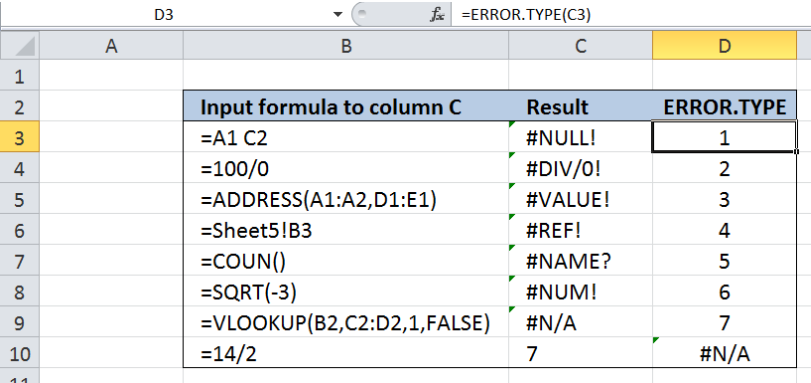Figure 1. Final result: Excel ERROR.TYPE function

Final formula:  `=ERROR.TYPE(C3)`

## Syntax of ERROR.TYPE Function

ERROR.TYPE function identifies an error value and returns a number that corresponds to the error value in Excel; otherwise, if no error exists, it returns the #N/A error

`=ERROR.TYPE(error_val)  `

Parameters:

• error_val  – the error value for which we want to find the ERROR.TYPE identifying number
• Below table shows the identifying number for every error value in Excel: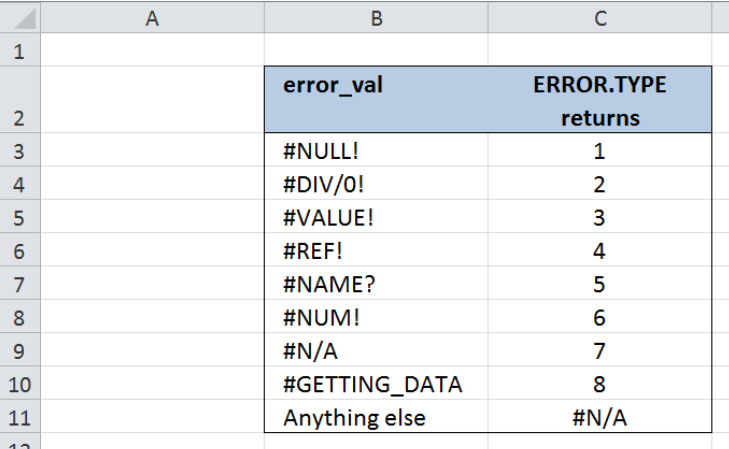Figure 2.  Error type values for specific error values in Excel

## Setting up Our Data

Our table consists of three columns:  Input formula to column C (column B), Result (column C) and ERROR.TYPE (column D).  Note that the contents in column B are inputted into column C in order to show some common errors in Excel.  We want to identify the type of error in column C and return the identifying number for each error value in column D.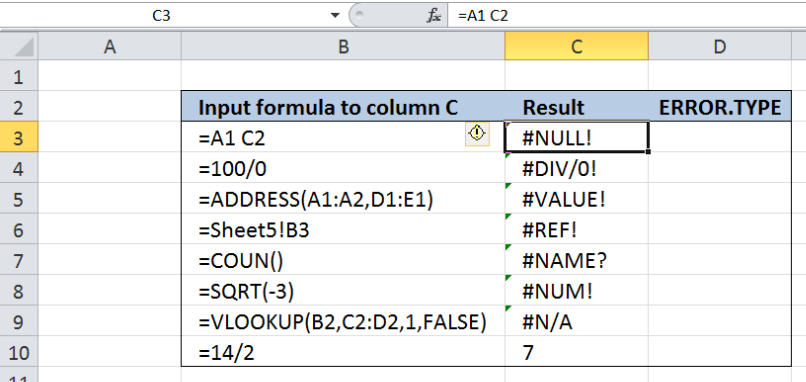Figure 3.  Sample data for Excel ERROR.TYPE function

## Identify the type of error using ERROR.TYPE

In order to identify the specific type of error in column B based on the error type values provided by the ERROR.TYPE function,  we follow these steps:

Step 1.  Select cell D3

Step 2.  Enter the formula: `=``ERROR.TYPE(C3)`

Step 3: Press ENTER

Step 4:  Copy the formula in cell D3 to cells D4:D10 by clicking the “+” icon at the bottom-right corner of cell D3 and dragging it down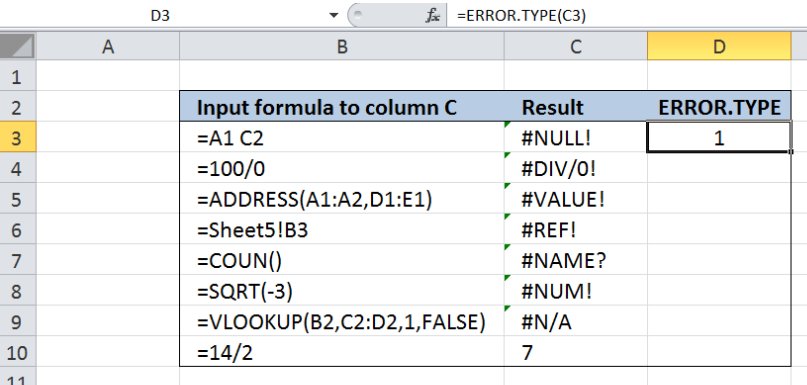Figure 4.  Entering the ERROR.TYPE function to identify the type of error

Figure 2 above shows the list of numbers from 1 to 8 which represents the error values in Excel.  Cell C3 has the error value #NULL! and based on Figure 2, #NULL! Is error type 1. As a result, our ERROR.TYPE function returns the value if “1” in cell D3.

The succeeding cells D4:D9 show the error type values representing the specific error values found in column C.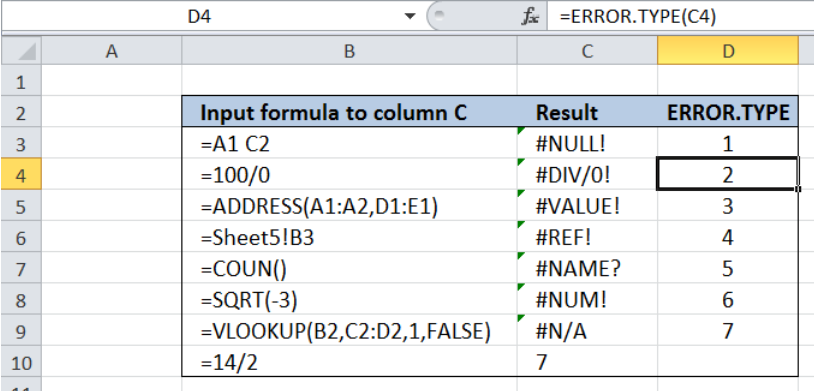Figure 5.  ERROR.TYPE identifying the type of error

For the last example in row 10, the value of C10 is the integer “7” which is not an error value.  Hence, our ERROR.TYPE formula returns the value #N/A in D10, which means that no error exists.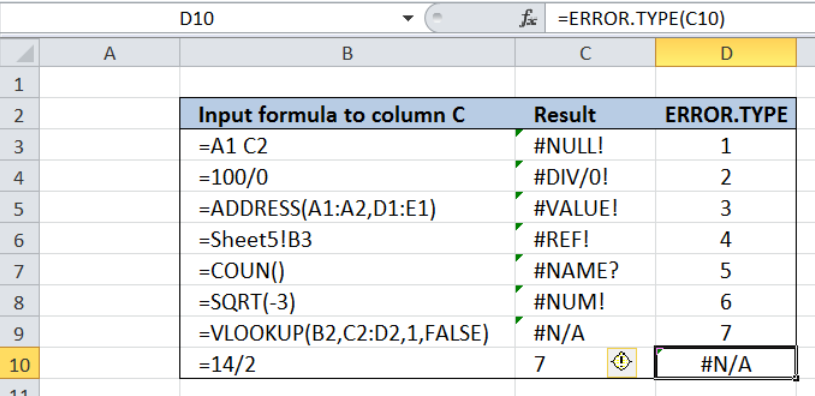Figure 6.  ERROR.TYPE returning #N/A for non-error values

Most of the time, the problem you will need to solve will be more complex than a simple application of a formula or function. If you want to save hours of research and frustration, try our live Excelchat service! Our Excel Experts are available 24/7 to answer any Excel question you may have. We guarantee a connection within 30 seconds and a customized solution within 20 minutes.

Solution examplesi want to hide a column but still use it in formula. is this even possible?
Solved by X. E. in 11 minsC5 contains 1625MPGW, F5 contains quantity. Need to search for MPGW and have quantity insert into column H5.
Solved by E. C. in 33 minsNeed help with lookup when you have multiple lookup values
Solved by V. J. in 20 minsI have a spread sheet with Items and sub Items. I would like to know if I can place a letter in another column it can get it to list the sub item number on another page in order
Solved by S. H. in 60 minsNeed to seperate text thats in a cell so i can sum them
Solved by G. C. in 10 mins